In this article, we will share MP Board Class 10th Maths Book Solutions Chapter 13 Surface Areas and Volumes Ex 13.1 Pdf, These solutions are solved subject experts from the latest edition books.

## MP Board Class 10th Maths Solutions Chapter 13 Surface Areas and Volumes Ex 13.1

Unless stated otherwise, take π = $$\frac{22}{7}$$

Question 1.
2 cubes each of volume 64 cm3 are joined end to end. Find the surface area of the resulting cuboid.
Solution:
Volume of each cube = 64 cm3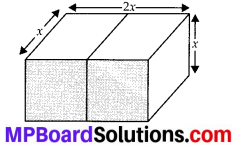Let the edge of each cube = x
∴ x3 = 64 cm3
⇒ x = 4 cm
Now, Length of the resulting cuboid (l) = 2x cm = 8 cm
Breadth of the resulting cuboid (b) = x cm = 4 cm
Height of the resulting cuboid (h) = x cm = 4 cm
∴ Surface area of the cuboid = 2 (lb + bh + hl)
= 2 [(8 × 4) + (4 × 4) + (4 × 8)] cm2
= 2 [32 + 16 + 32] cm2 = 2  cm2
= 160 cm2.Question 2.
A vessel is in the form of a hollow hemisphere mounted by a hollow cylinder. The diameter of the hemisphere is 14 cm and the total height of the vessel is 13 cm. Find the inner surface area of the vessel.
Solution:
For hemispherical part,
radius (r)= $$\frac{14}{2}$$ = 7cm
∴ Curved surface area = 2πr2
= 2 × $$\frac{22}{7}$$ × 7 × 7cm2
= 308cm2 7
Total height of vessel = 13 cm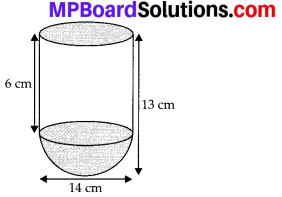∴ Height of cylinder = (13 – 7)cm = 6 cm and radius(r) = 7 cm
∴ Curved surface area of cylinder = 2πrh
= 2 × $$\frac{22}{7}$$ × 7 × 6cm2 = 264cm2 7
∴ Inner surface area of vessel = (308 + 264)cm2 = 572 cm2

Question 3.
A toy is in the form of a cone of radius 3.5 cm mounted on a hemisphere of same radius. The total height of the toy is 15.5 cm. Find the total surface area of the toy.
Solution:
Let h be the height of cone and r be the radius of cone and hemisphere.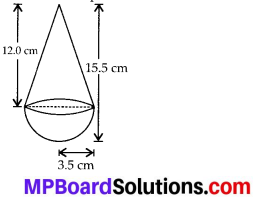∴ h = [height of toy – radius of hemi sphere]
= (15.5 – 3.5) cm = 12 cm
Also l2 = h2 + r2 = 122 + (3.5)2 = 156.25 cm2
∴ l = 12.5 cm
Curved surface area of the conical part = πrl
Curved surface area of the hemispherical part = 2πr2
∴ Total surface area of the toy = πrl + 2πr2
= πr(l + 2 r)
= $$\frac{22}{7} \times \frac{35}{10}$$ (12.5 + 2 × 3.5) cm2
= 11 × (12.5 + 7) cm2 = 11 × 19.5 cm2
= 214.5 cm2Question 4.
A cubical block of side 7 cm is surmounted by a hemisphere. What is the greatest diameter the hemisphere can have? Find the surface area of the solid.
Solution:
Let side of the block, l = 7 cm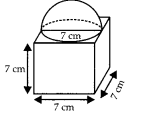∴ The greatest diameter of the hemisphere = 7 cm
Surface area of the solid = [Total surface area of the cubical block] + [C.S.A. of the hemisphere] – [Base area of the hemisphere]
= 6 × l2 + 2πr2 – πr2
[where l = 7 cm and r = $$\frac{7}{2}$$ cm]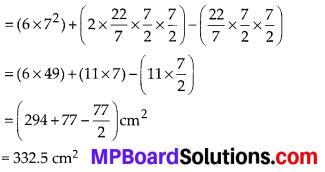Question 5.
A hemispherical depression is cut out from one face of a cubical wooden block such that the diameter l of the hemisphere is equal to the edge of the cube. Determine the surface area of the remaining solid.
Solution:
Let l be the side of the cube.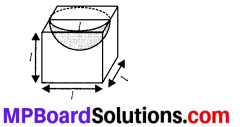∴ Diameter of the hemisphere = l
⇒ Radius of the hemisphere (r) = $$\frac{l}{2}$$
Curved surface area of hemisphere = 2πr2
= 2 × π × $$\frac{l}{2} \times \frac{l}{2}=\frac{\pi l^{2}}{2}$$
Base area of the hemisphere = πr2
= $$\pi\left(\frac{l}{2}\right)^{2}=\frac{\pi l^{2}}{4}$$
Surface area of the cube = 6 × l2 = 6l2
∴ Surface area of the remaining solid = [Total surface area of cube + C.S.A. of hemisphere – base area of hemisphere]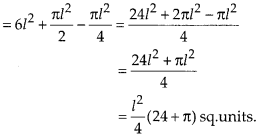Question 6.
A medicine capsule is in the shape of a cylinder with two hemispheres stuck to each of its ends (see figure). The length of the entire capsule is 14 mm and the diameter of the capsule is 5 mm. Find its surface area.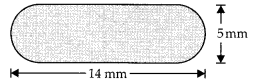Solution:
Radius of the hemispherical part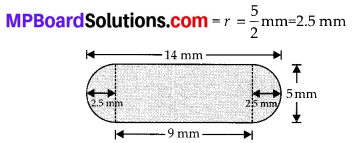Curved surface area of one hemispherical part = 2πr2
∴ Surface area of both hemispherical parts
= 2(2πr2) = 4πr2 = [4 × $$\frac{22}{7} \times\left(\frac{25}{10}\right)^{2}$$] mm2
= $$\left(4 \times \frac{22}{7} \times \frac{25}{10} \times \frac{25}{10}\right)$$ mm2
Entire length of capsule = 14 mm
∴ Length of cylindrical part = [Length of capsule – Radius of two hemispherical part]
= (14 – 2 × 2.5)mm = 9mm Area of cylindrical part = 2πrh
= (2 × $$\frac{22}{7}$$ × 2.5 × 9 ]mm2 = (2 × $$\frac{22}{7} \times \frac{25}{10}$$ × 9) mm2
Total surface area
= [Surface area of cylindrical part + Surface area of both hemispherical parts]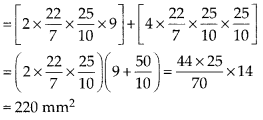Question 7.
A tent is in the shape of a cylinder surmounted by a conical top. If the height and diameter of the cylindrical part are 2.1 m and 4 m respectively, and the slant height of the top is 2.8 m, find the area of the canvas used for making the tent. Also, find the cost of the canvas of the tent at the rate of ₹ 500 per m2. (Note that the base of the tent will not be covered with canvas.)
Solution: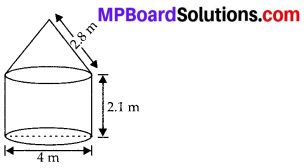For cylindrical part:
Radius (r) = $$\frac{4}{2}$$ m = 2m and height (h) = 2.1 m
∴ Curved surface area = 2πrh = (2 × $$\frac{22}{7}$$ × 2 × $$\frac{21}{10}$$)m2
For conical part:
Slant height (l) = 2.8 m
and base radius (r) = 2 m
∴ Curved surface area
= πrl = ($$\frac{22}{7}$$ × 2 × $$\frac{28}{10}$$) m2
∴ Total surface area = [Curved surface area of the cylindrical part] + [Curved surface area of conical part]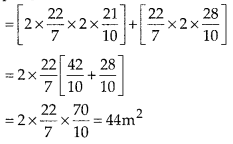Cost of the canvas used :
Cost of 1 m2 of canvas = ₹ 500
∴ Cost of 44 m2 of canvas = ₹ (500 × 44)
= ₹ 22000.

Question 8.
From a solid cylinder whose height is 2.4 cm and diameter 1.4 cm, a conical cavity of the same height and same diameter is hollowed out. Find the total surface area of the remaining solid to the nearest cm2.
Solution: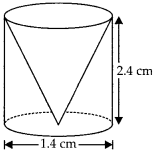For cylindrical part :
Height (h) = 2.4 cm and diameter = 1.4 cm
⇒ Radius (r) = 0.7 cm
∴ Total surface area of the cylindrical part
= 2πrh + 2πr2 = 2πr [h + r]
= 2 × $$\frac{22}{7} \times \frac{7}{10}$$ [2.4 + 0.7]
= $$\frac{44}{10}$$ × 3.1 = $$\frac{44 \times 31}{100}$$ = $$\frac{1364}{100}$$ cm2
For conical part:
Base radius (r) = 0.7 cm and height (h) = 2.4 cm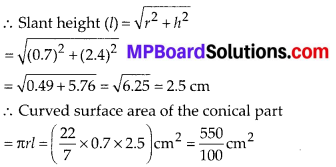Base area of the conical part
= $$\pi r^{2}=\frac{22}{7} \times\left(\frac{7}{10}\right)^{2}=\frac{22 \times 7}{100} \mathrm{cm}^{2}=\frac{154}{100} \mathrm{cm}^{2}$$
Total surface area of the remaining solid = [(Total surface area of cylindrical part) + (Curved surface area of conical part) – (Base area of the conical part)]
= $$\left[\frac{1364}{100}+\frac{550}{100}-\frac{154}{100}\right] \mathrm{cm}^{2}=\frac{1760}{100} \mathrm{cm}^{2}$$
Hence, total surface area to the nearest cm2 is 18cm2.Question 9.
A wooden article was made by scooping out a hemisphere from each end of a solid cylinder, as shown in figure. If the height of the cylinder is 10 cm, and its base is of radius 3.5 cm, find the total surface area of the article.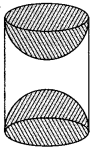Solution:
Radius of the cylinder (r) = 3.5 cm
Height of the cylinder (h) = 10 cm
∴ Curved surface area = 2πrh
= 2 × $$\frac{22}{7} \times \frac{35}{10}$$ × 10cm2 = 220cm2
Curved surface area of a hemisphere = 2πr2
∴ Curved surface area of both hemispheres
= 2 × 2πr2 = 4πr2 = 4 × $$\frac{22}{7} \times \frac{35}{10} \times \frac{35}{10}$$ cm2
= 154 cm2
Total surface area of the remaining solid = (220 + 154) cm2 = 374 cm2.